# A sound source $S$ is moving along a straight track with speed $v$,`
Question:

A sound source $S$ is moving along a straight track with speed $v$, and is emitting sound of frequency $v_{\mathrm{o}}$ (see figure). An observer is standing at a finite distance, at the point $O$, from the track. The time variation of frequency heard by the observer is best represented by:

$\left(t_{0}\right.$ represents the instant when the distance between the source and observer is minimum)

1.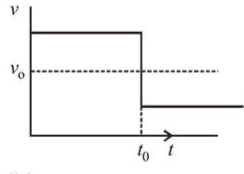2.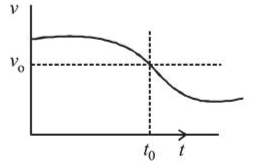3.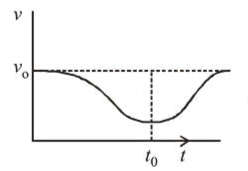4.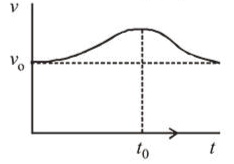Correct Option: , 2

Solution:

(2) Frequency heard by the observer

$v_{\text {observed }}=\left(\frac{v_{\text {sound }}}{\mathrm{v}_{\text {sound }}-v \cos \theta}\right) v_{0}$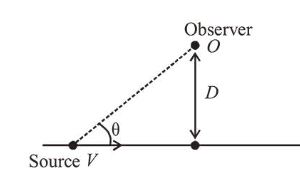Initially $\theta$ will be less so $\cos \theta$ more.

$\therefore v_{\text {observed }}$ more, then it will decrease.